Open In App

# Top 50 C Programming Interview Questions and Answers

At Bell Labs, Dennis Ritchie developed the C programming language between 1971 and 1973. C is a mid-level structured-oriented programming and general-purpose programming. It is one of the old and most popular programming languages. There are many applications in which C programming language is used, including language compilers, operating systems, assemblers, network drivers, text editors, print spoolers, modern applications, language interpreters, databases, and utilities.## C Programming Interview Questions

C Programming language is one of the languages that are both complex yet important to learn for strengthening your programming skills. Interview questions can be categorized into two parts:

1. For Freshers
2. For Experienced

## C Programming Interview Questions – For Freshers

### 1. Why is C called a mid-level programming language?

Due to its ability to support both low-level and high-level features, C is considered a middle-level language. It is both an assembly-level language, i.e. a low-level language, and a higher-level language. Programs that are written in C are converted into assembly code, and they support pointer arithmetic (low-level) while being machine-independent (high-level). Therefore, C is often referred to as a middle-level language. C can be used to write operating systems and menu-driven consumer billing systems.

### 2. What are the features of the C programming language?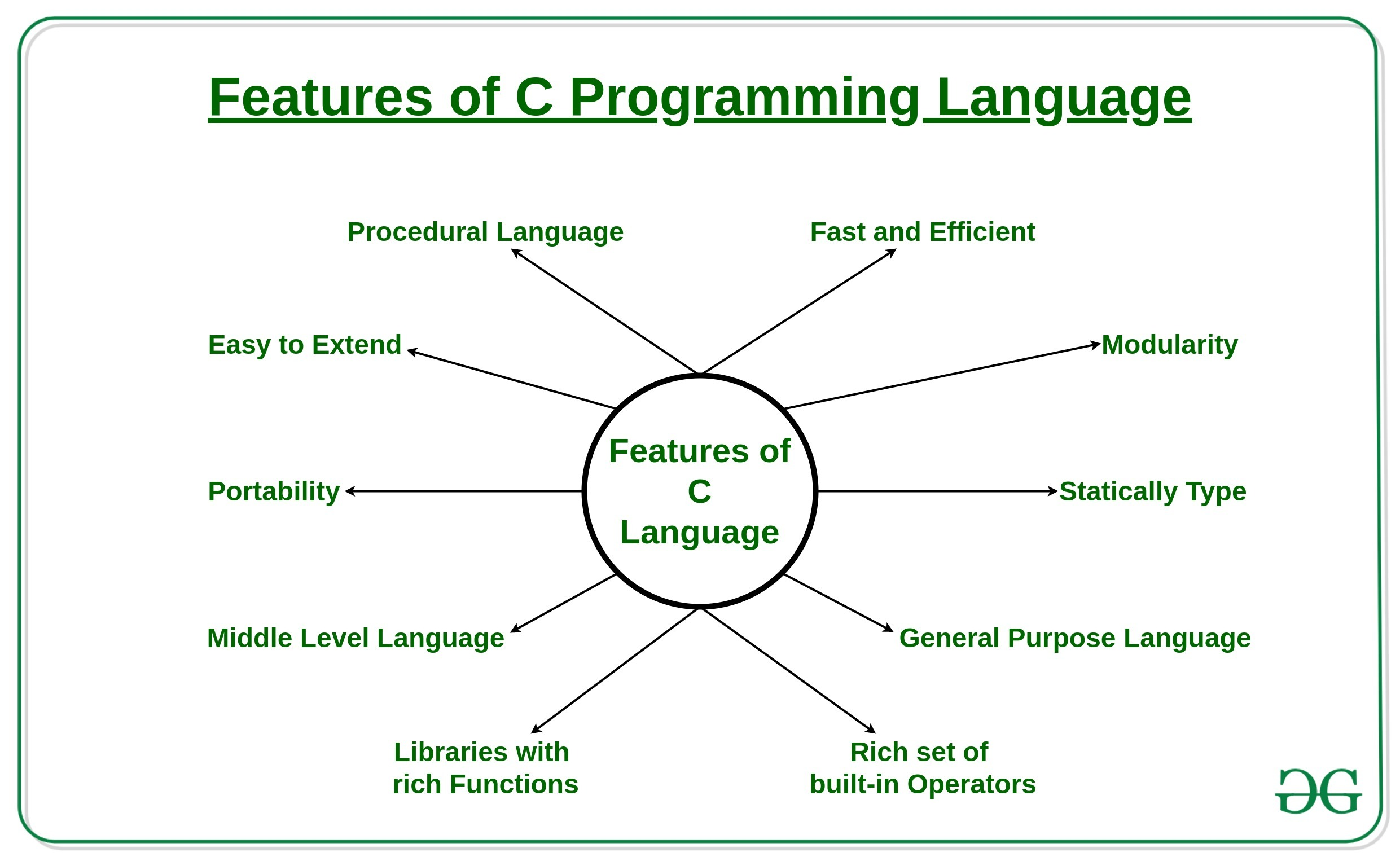Features of C Programming language

For more information, refer to the article – Features of C programming language.

### 3. What are basic data types supported in the C Programming Language?

Each variable in C has an associated data type. Each data type requires different amounts of memory and has some specific operations which can be performed over it. It specifies the type of data that the variable can store like integer, character, floating, double, etc. In C data types are broadly classified into 4 categories:

• Primitive data types: Primitive data types can be further classified into integer, and floating data types.
• Void Types: Void data types come under primitive data types. Void data types provide no result to their caller and have no value associated with them.
• User Defined data types: These data types are defined by the user to make the program more readable.
• Derived data types: Data types that are derived from primitive or built-in data types.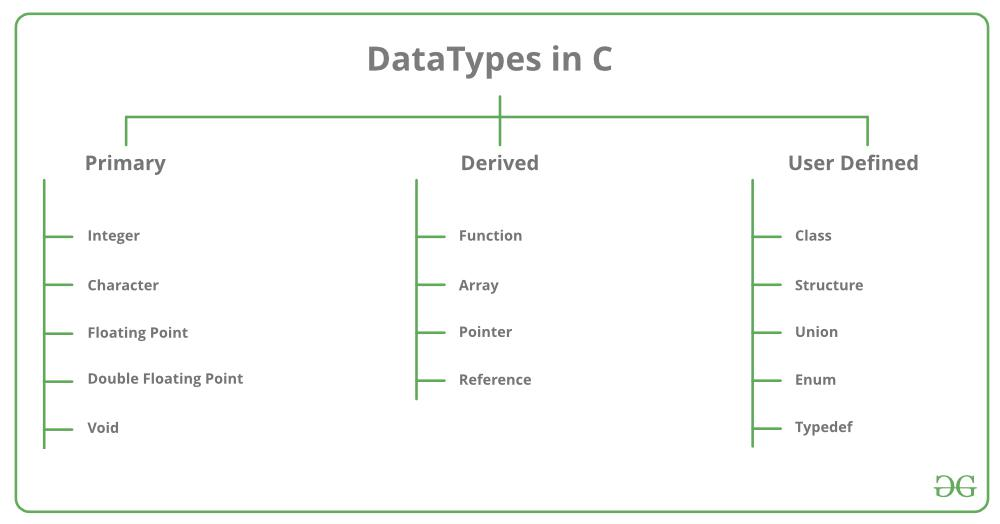Data Types in C

For more information, refer to the article – Data Types in C.

### 4. What are tokens in C?

Tokens are identifiers or the smallest single unit in a program that is meaningful to the compiler. In C we have the following tokens:

• Keywords: Predefined or reserved words in the C programming language. Every keyword is meant to perform a specific task in a program. C Programming language supports 32 keywords.
• Identifiers: Identifiers are user-defined names that consist of an arbitrarily long sequence of digits or letters with either a letter or the underscore (_) as a first Character. Identifier names can’t be equal to any reserved keywords in the C programming language. There are a set of rules which a programmer must follow in order to name an identifier in C.
• Constants: Constants are normal variables that cannot be modified in the program once they are defined. Constants refer to a fixed value. They are also referred to as literals.
• Strings: Strings in C are an array of characters that end with a null character (‘\0). Null character indicates the end of the string;
• Special Symbols: Some special symbols in C have some special meaning and thus, they cannot be used for any other purpose in the program. # = {} () , * ; [] are the special symbols in C programming language.
• Operators: Symbols that trigger an action when they are applied to any variable or any other object. Unary, Binary, and ternary operators are used in the C Programming language.

### 5. What do you mean by the scope of the variable?

Scope in a programming language is the block or a region where a defined variable will have its existence and beyond that region, the variable is automatically destroyed.  Every variable has its defined scope. In simple terms, the scope of a variable is equal to its life in the program. The variable can be declared in three places These are:

• Local Variables: Inside a given function or a block
• Global Variables: Out of all functions globally inside the program.
• Formal Parameters: In-function parameters only.

### 6. What are preprocessor directives in C?

In C preprocessor directives are considered the built-in predefined functions or macros that act as a directive to the compiler and are executed before the program execution. There are multiple steps involved in writing and executing a program in C. Main types of Preprocessor Directives are Macros, File Inclusion, Conditional Compilation, and Other directives like #undef, #pragma, etc.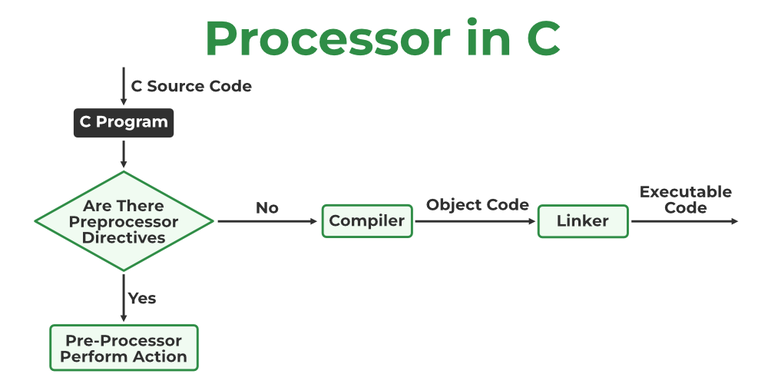Processor in C

For more information, refer to the article – Preprocessor Directives in C

### 7. What is the use of static variables in C?

Static variables in the C programming language are used to preserve the data values between function calls even after they are out of their scope. Static variables preserve their values in their scope and they can be used again in the program without initializing again. Static variables have an initial value assigned to 0 without initialization.

## C

 `// C program to print initial``// value of static variable``#include ``int` `main()``{``    ``static` `int` `var;``    ``int` `x;``    ``printf``(``"Initial value of static variable %d\n"``, var);``    ``printf``(``"Initial value of variable without static %d"``,``           ``x);``    ``return` `0;``}`

Output

```Initial value of static variable 0
Initial value of variable without static 0```

For more information, refer to the article – Static Variables in C

### 8. What is the difference between malloc() and calloc() in the C programming language?

calloc() and malloc() library functions are used to allocate dynamic memory. Dynamic memory is the memory that is allocated during the runtime of the program from the heap segment. “stdlib.h” is the header file that is used to facilitate dynamic memory allocation in the C Programming language.

For more information, refer to the article – Dynamic Memory Allocation in C using malloc(), calloc(), free(), and realloc()

### 9. What do you mean by dangling pointers and how are dangling pointers different from memory leaks in C programming?

Pointers pointing to deallocated memory blocks in C Programming are known as dangling pointers i.e, whenever a pointer is pointing to a memory location and In case the variable is deleted and the pointer still points to that same memory location then it is known as a dangling pointer variable.
In C programming memory leak occurs when we allocate memory with the help of the malloc() or calloc() library function, but we forget to free the allocated memory with the help of the free() library function. Memory leak causes the program to use an undefined amount of memory from the RAM which makes it unavailable for other running programs this causes our program to crash.

## C

 `// C program to convert number to``// string using sprintf()``#include ``#include `` ` `// Driver code``int` `main()``{``    ``char` `res;``    ``float` `a = 32.23;``    ``sprintf``(res, ``"%f"``, a);``    ``printf``(``"\nThe string for the num is %s"``, res);``    ``return` `0;``}`

Output

`The string for the num is 32.230000`

### 11. What is recursion in C?

Recursion is the process of making the function call itself directly or indirectly. A recursive function solves a particular problem by calling a copy of itself and solving smaller subproblems that sum up the original problems. Recursion helps to reduce the length of code and make it more understandable. The recursive function uses a LIFO ( Last In First Out ) structure like a stack. Every recursive call in the program requires extra space in the stack memory.

### 12. What is the difference between the local and global variables in C?

Local variables are declared inside a block or function but global variables are declared outside the block or function to be accessed globally.

### 13. What are pointers and their uses?

Pointers are used to store the address of the variable or a memory location. Pointer can also be used to refer to another pointer function. The main purpose of the pointer is to save memory space and increase execution time. Uses of pointers are:

• To pass arguments by reference
• For accessing array elements
• To return multiple values
• Dynamic memory allocation
• To implement data structures
• To do system-level programming where memory addresses are useful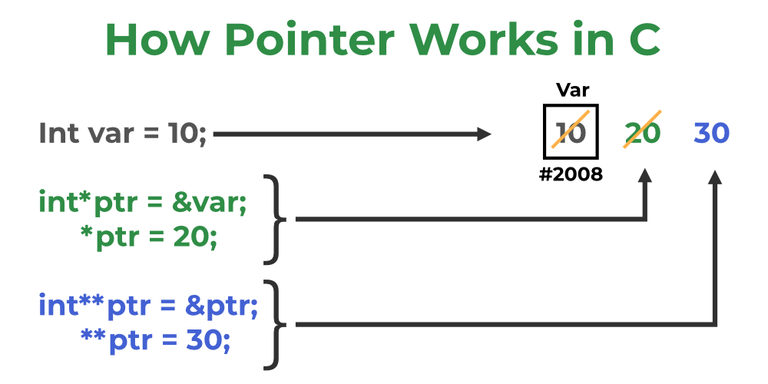Working of Pointer

For more information, refer to the article – Pointer Uses in C.

### 14. What is typedef in C?

In C programming, typedef is a keyword that defines an alias for an existing type. Whether it is an integer variable, function parameter, or structure declaration, typedef will shorten the name.

Syntax:

typedef <existing-type> <alias-name>

Here,

• existing type is already given a name.
• alias name is the new name for the existing variable.

Example:

`typedef long long ll`

### 15. What are loops and how can we create an infinite loop in C?

Loops are used to execute a block of statements repeatedly. The statement which is to be repeated will be executed n times inside the loop until the given condition is reached. There are two types of loops Entry controlled and Exit-controlled loops in the C programming language. An Infinite loop is a piece of code that lacks a functional exit. So, it repeats indefinitely. There can be only two things when there is an infinite loop in the program. One it was designed to loop endlessly until the condition is met within the loop. Another can be wrong or unsatisfied break conditions in the program.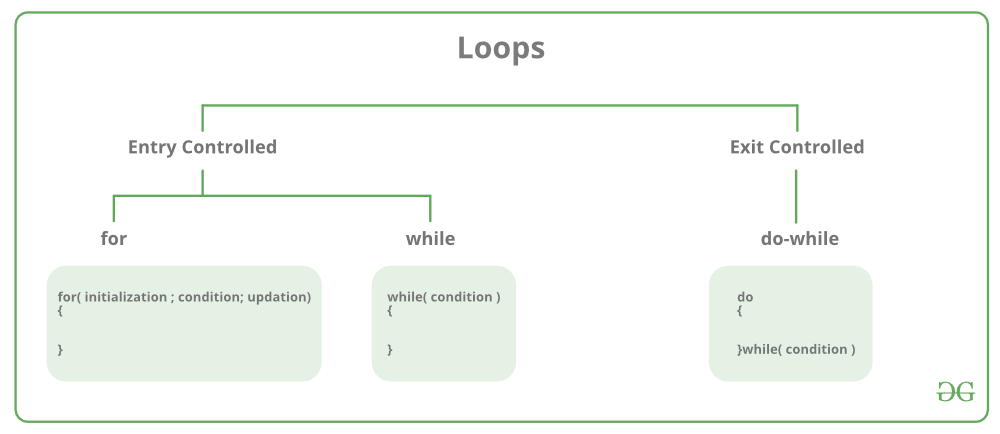Types of Loops

Below is the program for infinite loop in C:

## C

 `// C program for infinite loop``// using for, while, do-while``#include `` ` `// Driver code``int` `main()``{``    ``for` `(;;) {``        ``printf``(``"Infinite-loop\n"``);``    ``}`` ` `    ``while` `(1) {``        ``printf``(``"Infinite-loop\n"``);``    ``}`` ` `    ``do` `{``        ``printf``(``"Infinite-loop\n"``);``    ``} ``while` `(1);`` ` `    ``return` `0;``}`

### 16. What is the difference between type casting and type conversion?

For more information, refer to the article – Type Casting and Type Conversion.

### 17. What are header files and their uses?

C language has numerous libraries which contain predefined functions to make programming easier. Header files contain predefined standard library functions. All header files must have a ‘.h’ extension. Header files contain function definitions, data type definitions, and macros which can be imported with the help of the preprocessor directive ‘#include’. Preprocessor directives instruct the compiler that these files are needed to be processed before the compilation.
There are two types of header files i.e, User-defined header files and Pre-existing header files. For example, if our code needs to take input from the user and print desired output to the screen then ‘stdio.h’ header file must be included in the program as #include<stdio.h>. This header file contains functions like scanf() and printf() which are used to take input from the user and print the content.

### 18. What are the functions and their types?

The function is a block of code that is used to perform a task multiple times rather than writing it out multiple times in our program. Functions avoid repetition of code and increase the readability of the program. Modifying a program becomes easier with the help of function and hence reduces the chances of error. There are two types of functions:

• User-defined Functions: Functions that are defined by the user to reduce the complexity of big programs. They are built only to satisfy the condition in which the user is facing issues and are commonly known as “tailor-made functions”.
• Built-in Functions: Library functions are provided by the compiler package and consist of special functions with special and different meanings. These functions give programmers an edge as we can directly use them without defining them.

### 19. What is the difference between macro and functions?

A macro is a name that is given to a block of C statements as a pre-processor directive. Macro is defined with the pre-processor directive. Macros are pre-processed which means that all the macros would be preprocessed before the compilation of our program. However, functions are not preprocessed but compiled.

## C Programming Interview Questions – Intermediate Level

### 20. How to convert a string to numbers in C?

In C we have 2 main methods to convert strings to numbers i.e, Using string stream, Using stoi() library Function, and using atoi() library function.

• sscanf(): It reads input from a string rather than standard input.
• atoi(): This function takes a string literal or a character array as an argument and an integer value is returned.

For more information, refer to the article – String to Numbers in C

### 21. What are reserved keywords?

Every keyword is meant to perform a specific task in a program. Their meaning is already defined and cannot be used for purposes other than what they are originally intended for. C Programming language supports 32 keywords. Some examples of reserved keywords are auto, else, if, long, int, switch, typedef, etc.

For more information, refer to the article – Variables and Keywords in C

### 22. What is a structure?

The structure is a keyword that is used to create user-defined data types. The structure allows storing multiple types of data in a single unit. The structure members can only be accessed through the structure variable.

Example:

struct student
{
char name;
int roll_no;
char branch;
};

Below is the C program to implement structure:

## C

 `// C Program to show the``// use of structure``#include ``#include `` ` `// Structure student declared``struct` `student {``    ``char` `name;``    ``int` `roll_no;``    ``char` `address;``    ``char` `branch;``};`` ` `// Driver code``int` `main()``{``    ``struct` `student obj;`` ` `    ``strcpy``(obj.name, ``"Kamlesh_Joshi"``);``    ``obj.roll_no = 27;``    ``strcpy``(obj.address, ``"Haldwani"``);``    ``strcpy``(obj.branch, ``"Computer Science And Engineering"``);`` ` `    ``// Accessing members of student obj``    ``printf``(``"Name: %s\n"``, obj.name);``    ``printf``(``"Roll_No: %d \n"``, obj.roll_no);``    ``printf``(``"Address: %s\n"``, obj.address);``    ``printf``(``"Branch: %s"``, obj.branch);`` ` `    ``return` `0;``}`

Output

```Name: Kamlesh_Joshi
Roll_No: 27
Branch: Computer Science And Engineering```

### 23. What is union?

A union is a user-defined data type that allows users to store multiple types of data in a single unit. However, a union does not occupy the sum of the memory of all members. It holds the memory of the largest member only. Since the union allocates one common space for all the members we can access only a single variable at a time. The union can be useful in many situations where we want to use the same memory for two or more members.

Syntax:

union name_of_union
{
data_type name;
data_type name;
};

### 24. What is an r-value and value?

An “l-value” refers to an object with an identifiable location in memory (i.e. having an address).  An “l-value” will appear either on the right or left side of the assignment operator(=). An “r-value” is a data value stored in memory at a given address. An “r-value” refers to an object without an identifiable location in memory (i.e. without an address). An “r-value” is an expression that cannot be assigned a value, therefore it can only exist on the right side of an assignment operator (=).

Example:

int val = 20;

Here, val is the ‘l-value’, and 20 is the ‘r-value’.

For more information, refer to the article – r-value and l-value in C

### 25. What is the difference between call by value and call by reference?

For more information, refer to the article – Call by Value and Call by Reference

### 26. What is the sleep() function?

sleep() function in C allows the users to wait for a current thread for a given amount of time. sleep() function will sleep the present executable for the given amount of time by the thread but other operations of the CPU will function properly. sleep() function returns 0 if the requested time has elapsed.

For more information, refer to the article – sleep() Function in C

### 27. What are enumerations?

In C, enumerations (or enums) are user-defined data types. Enumerations allow integral constants to be named, which makes a program easier to read and maintain. For example, the days of the week can be defined as an enumeration and can be used anywhere in the program.

`enum enumeration_name{constant1, constant2, ... };`

## C

 `// An example program to demonstrate working``// of enum in C``#include `` ` `enum` `week { Mon, Tue, Wed, Thur, Fri, Sat, Sun };`` ` `int` `main()``{``    ``enum` `week day;``    ``day = Wed;``    ``printf``(``"%d"``, day);``    ``return` `0;``}`

Output

`2`

In the above example, we declared “day” as the variable, and the value of “Wed” is allocated to day, which is 2. So as a result, 2 is printed.

For more information, refer to the article – Enumeration (or enum) in C

### 28: What is a volatile keyword?

Volatile keyword is used to prevent the compiler from optimization because their values can’t be changed by code that is outside the scope of current code at any time. The System always reads the current value of a volatile object from the memory location rather than keeping its value in a temporary register at the point it is requested, even if previous instruction is asked for the value from the same object.

### 29. Write a C program to print the Fibonacci series using recursion and without using recursion.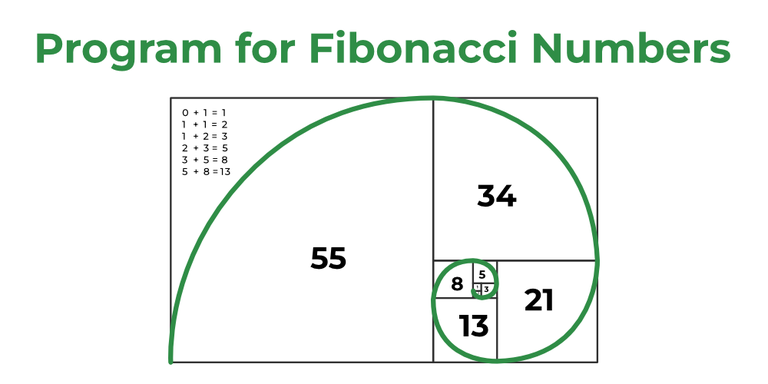Fibonacci Numbers

## C

 `// C program to print Fibonacci Series``// with recursion and without recursion``#include `` ` `// Recursive Function to print``// Fibonacci Series``void` `Fibonacci(``int` `num, ``int` `first, ``int` `second, ``int` `third)``{``    ``if` `(num > 0) {``        ``third = first + second;``        ``first = second;``        ``second = third;`` ` `        ``printf``(``"%d "``, third);`` ` `        ``// Recursive call for it's``        ``// n-1 value``        ``Fibonacci(num - 1, first, second, third)``    ``}``}`` ` `// Driver code``int` `main()``{``    ``int` `num;`` ` `    ``printf``(``"Please Enter number of Elements: "``);``    ``scanf``(``"%d"``, &num);`` ` `    ``printf``(``        ``"Fibonacci Series with the help of Recursion:\n"``);`` ` `    ``printf``(``"%d %d "``, 0, 1);`` ` `    ``// we are passing n-2 because we have``    ``// already printed 2 numbers i.e, 0 and 1`` ` `    ``Fibonacci(num - 2, 0, 1, 0);`` ` `    ``printf``(``"\nFibonacci Series without Using Recursion:\n"``);`` ` `    ``int` `first = 0, second = 1, third = 0;`` ` `    ``printf``(``"%d %d "``, 0, 1);`` ` `    ``// Loop will start from 2 because we have``    ``// already printed 0 and 1``    ``for` `(``int` `i = 2; i < num; i++) {``        ``third = first + second;`` ` `        ``printf``(``"%d "``, third);`` ` `        ``first = second;``        ``second = third;``    ``}`` ` `    ``return` `0;``}`

```Output:
Please Enter number of Elements: 5
Fibonacci Series with the help of Recursion:
0 1 1 2 3
Fibonacci Series without Using Recursion:
0 1 1 2 3 ```

### 30. Write a C program to check whether a number is prime or not.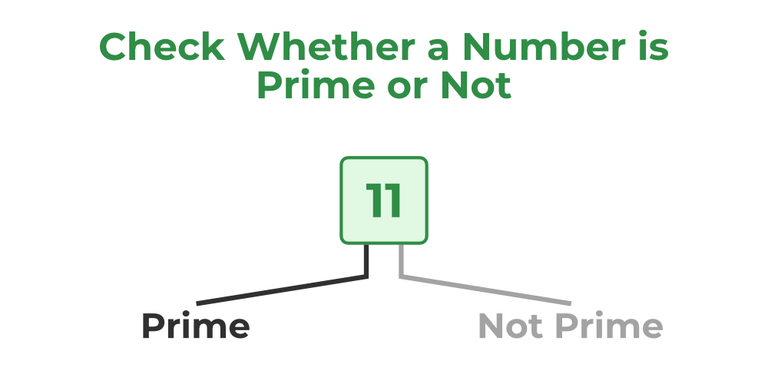Number Prime or not

## C

 `// C program to check if a``// number is prime``#include ``#include `` ` `// Driver code``int` `main()``{``    ``int` `num;``    ``int` `check = 1;`` ` `    ``printf``(``"Enter a number: \n"``);``    ``scanf``(``"%d"``, &num);`` ` `    ``// Iterating from 2 to sqrt(num)``    ``for` `(``int` `i = 2; i <= ``sqrt``(num); i++) {``        ``// If the given number is divisible by``        ``// any number between 2 and n/2 then``        ``// the given number is not a prime number``        ``if` `(num % i == 0) {``            ``check = 0;``            ``break``;``        ``}``    ``}`` ` `    ``if` `(num <= 1) {``        ``check = 0;``    ``}`` ` `    ``if` `(check == 1) {``        ``printf``(``"%d is a prime number"``, num);``    ``}``    ``else` `{``        ``printf``(``"%d is not a prime number"``, num);``    ``}`` ` `    ``return` `0;``}`

### 31. How is source code different from object code?

For more information, refer to the article – Source vs Object Code.

### 32. What is static memory allocation and dynamic memory allocation?

• Static memory allocation: Memory allocation which is done at compile time is known as static memory allocation. Static memory allocation saves running time. It is faster than dynamic memory allocation as memory allocation is done from the stack. This memory allocation method is less efficient as compared to dynamic memory allocation. It is mostly preferred in the array.
• Dynamic memory allocation: Memory allocation done at execution or run time is known as dynamic memory allocation. Dynamic memory allocation is slower than static memory allocation as memory allocation is done from the heap. This memory allocation method is more efficient as compared to static memory allocation. It is mostly preferred in the linked list.

For more information, refer to the article – Static and Dynamic Memory Allocation in C

### 33. What is pass-by-reference in functions?

Pass by reference allows a function to modify a variable without making a copy of the variable. The Memory location of the passed variable and parameter is the same, so any changes done to the parameter will be reflected by the variables as well.

## C

 `// C program to change a variable``// using pass by reference``#include `` ` `// * used to dereference the variable``void` `change(``int``* num)``{``    ``// value of num changed to 30``    ``*num = 30;``}`` ` `// Driver code``int` `main()``{``    ``int` `num = 20;``    ``printf``(``"Value of num before passing is: %d\n"``, num);`` ` `    ``// Calling change function by passing address``    ``change(&num);`` ` `    ``printf``(``"Value of num after changing with the help of "``           ``"function is: %d"``,``           ``num);`` ` `    ``return` `0;``}`

### 34. What is a memory leak and how to avoid it?

Whenever a variable is defined some amount of memory is created in the heap. If the programmer forgets to delete the memory. This undeleted memory in the heap is called a memory leak. The Performance of the program is reduced since the amount of available memory was reduced. To avoid memory leaks, memory allocated on the heap should always be cleared when it is no longer needed.

### 35. What are command line arguments?

Arguments that are passed to the main() function of the program in the command-line shell of the operating system are known as command-line arguments.

Syntax:

int main(int argc, char *argv[]){/*code which is to be executed*/}

For more information, refer to the article – Command Line Arguments in C

### 36. What is an auto keyword?

Every local variable of a function is known as an automatic variable in the C language. Auto is the default storage class for all the variables which are declared inside a function or a block. Auto variables can only be accessed within the block/function they have been declared. We can use them outside their scope with the help of pointers. By default auto keywords consist of a garbage value.

For more information, refer to the article – Storage Classes in C

## C

 `// C program to print hello-world``// without using semicolon``#include `` ` `// Driver code``int` `main()``{``    ``// This will print the hello-world``    ``// on the screen without giving any error``    ``if` `(``printf``(“Hello - World”)) {``    ``}``    ``return` `0;``}`

For more information, refer to the article – Hello World in C

### 38. Write a C program to swap two numbers without using a third variable.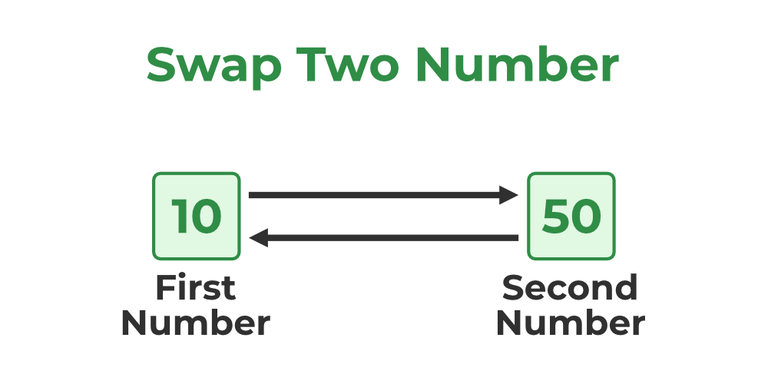Swap Two Number

## C

 `// C program to swap two variables``// without using a third variable``#include `` ` `int` `main()``{``    ``// Variable declaration``    ``int` `var1 = 50;``    ``int` `var2 = 60;`` ` `    ``printf``(``        ``"Values before swap are var1 = %d and var2 = %d\n"``,``        ``var1, var2);`` ` `    ``// var1 = 110 ( 50 + 60) ``    ``var1 = var1 + var2;`` ` `    ``// var2 = 50 (110 - 50)``    ``var2 = var1 - var2;`` ` `    ``// var1 = 60 (110 - 50)``    ``var1 = var1 - var2;`` ` `    ``printf``(``"Values after swap are var1 = %d  and var2 = %d"``,``           ``var1, var2);`` ` `    ``return` `0;``}`

Output

```Values before swap are var1 = 50 and var2 = 60
Values after swap are var1 = 60  and var2 = 50```

## C

 `// C program to check whether a``// string is palindrome or not.``#include ``#include `` ` `// Palindrome function to check``// whether a string is palindrome``// or not``void` `Palindrome(``char` `s[])``{``    ``// Start will start from 0th index``    ``// and end will start from length-1 ``    ``int` `start = 0;``    ``int` `end = ``strlen``(s) - 1;`` ` `    ``// Comparing characters until they``    ``// are same ``    ``while` `(end > start) {``        ``if` `(s[start++] != s[end--]) {``            ``printf``(``"%s is not a Palindrome \n"``, s);``            ``return``;``        ``}``    ``}``    ``printf``(``"%s is a Palindrome \n"``, s);``}`` ` `// Driver code``int` `main()``{``    ``Palindrome(``"abba"``);``    ``return` `0;``}`

Output

`abba is a Palindrome `

### 40. Explain modifiers.

Modifiers are keywords that are used to change the meaning of basic data types in C language. They specify the amount of memory that is to be allocated to the variable. There are five data type modifiers in the C programming language:

• long
• short
• signed
• unsigned
• long long

## C Programming Interview Questions – For Experienced

### 41. Write a program to print the factorial of a given number with the help of recursion.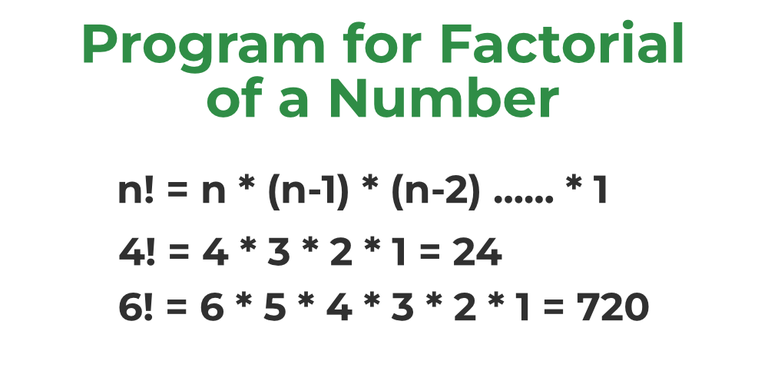Factorial of a Number

## C

 `// C program to find factorial``// of a given number``#include `` ` `// Function to find factorial of``// a given number``unsigned ``int` `factorial(unsigned ``int` `n)``{``    ``if` `(n == 0)``        ``return` `1;``    ``return` `n * factorial(n - 1);``}`` ` `// Driver code``int` `main()``{``    ``int` `num = 5;``    ``printf``(``"Factorial of %d is %d"``, num, factorial(num));``    ``return` `0;``}`

Output

`Factorial of 5 is 120`

## C

 `// C program to determine whether``// the given number is Armstrong``// or not``#include `` ` `// Driver code``int` `main()``{``    ``int` `n;`` ` `    ``printf``(``"Enter Number \n"``);``    ``scanf``(``"%d"``, &n);`` ` `    ``int` `var = n;``    ``int` `sum = 0;`` ` `    ``// Loop to calculate the order of``    ``// the given number ``    ``while` `(n > 0) {``        ``int` `rem = n % 10;``        ``sum = (sum) + (rem * rem * rem);``        ``n = n / 10;``    ``}`` ` `    ``// If the order of the number will be``    ``// equal to the number then it is``    ``// Armstrong number.``    ``if` `(var == sum) {``        ``printf``(``"%d is an Armstrong number \n"``, var);``    ``}``    ``else` `{``        ``printf``(``"%d is not an Armstrong number"``, var);``    ``}``    ``return` `0;``}`

Output

```Enter Number
0 is an Armstrong number ```

## C

 `// C program to reverse digits``// of a number``#include `` ` `// Driver code``int` `main()``{``    ``int` `n, rev = 0;`` ` `    ``printf``(``"Enter Number to be reversed : "``);``    ``scanf``(``"%d"``, &n);`` ` `    ``// r will store the remainder while we``    ``// reverse the digit and store it in rev``    ``int` `r = 0;``    ``  ``while` `(n != 0)``    ``{``        ``r = n % 10;``        ``rev = rev * 10 + r;``        ``n /= 10;``    ``}`` ` `    ``printf``(``"Number After reversing digits is: %d"``, rev);`` ` `    ``return` `0;``}`

Output:

```Enter Number to be reversed :
Number After reversing digits is: 321```

### 44. What is the use of an extern storage specifier?

The extern keyword is used to extend the visibility of the C variables and functions in the C language. Extern is the short name for external. It is used when a particular file needs to access a variable from any other file. Extern keyword increases the redundancy and variables with extern keyword are only declared not defined. By default functions are visible throughout the program, so there is no need to declare or define extern functions.

### 45. What is the use of printf() and scanf() functions in C Programming language? Also, explain format specifiers.

printf() function is used to print the value which is passed as the parameter to it on the console screen.

Syntax:

print(“%X”,variable_of_X_type);

scanf() method, reads the values from the console as per the data type specified.

Syntax:

scanf(“%X”,&variable_of_X_type);

In C format specifiers are used to tell the compiler what type of data will be present in the variable during input using scanf() or output using print().

• %c: Character format specifier used to display and scan character.
• %d, %i: Signed Integer format specifier used to print or scan an integer value.
• %f, %e, or %E: Floating-point format specifiers are used for printing or scanning float values.
• %s: This format specifier is used for String printing.
• %p: This format specifier is used for Address Printing.

For more information, refer to the article – Format Specifier in C

### 46. What is near, far, and huge pointers in C?

• Near Pointers: Near pointers are used to store 16-bit addresses only. Using the near pointer, we can not store the address with a size greater than 16 bits.
• Far Pointers: A far pointer is a pointer of 32 bits size. However, information outside the computer’s memory from the current segment can also be accessed.
• Huge Pointers: Huge pointer is typically considered a pointer of 32 bits size. But bits located outside or stored outside the segments can also be accessed.

### 47. Mention file operations in C.

In C programming Basic File Handling Techniques provide the basic functionalities that programmers can perform against the system.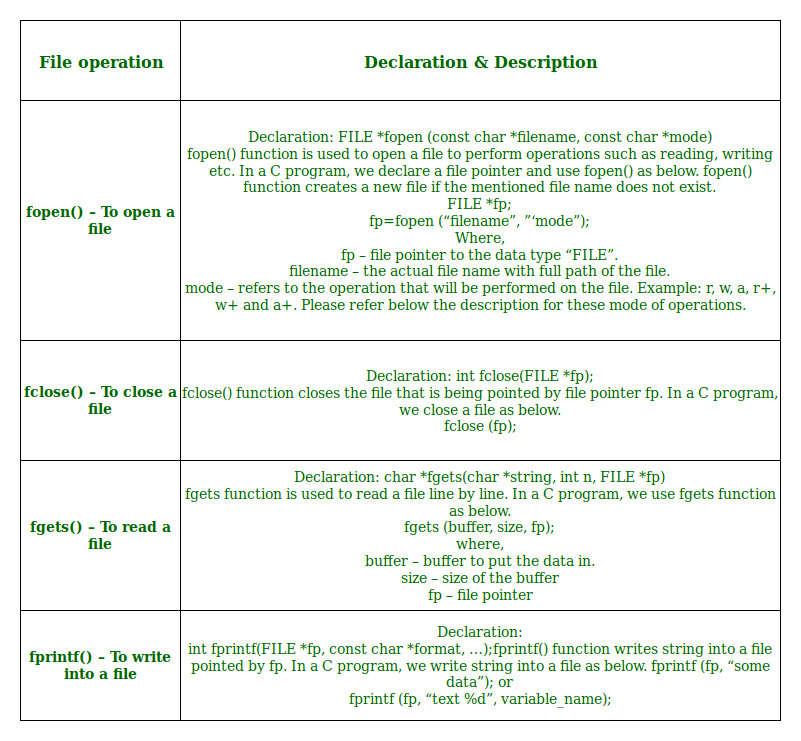File Operations in C

## C

 `// C program to check if the linked list is circular``#include ``#include ``struct` `Node {``    ``int` `data;``    ``struct` `Node* next;``};``int` `isCircular(``struct` `Node* head)``{`` ` `    ``// If given linked list is null then it is circular``    ``if` `(head == NULL) {``        ``return` `1;``    ``}``    ``struct` `Node* ptr;``    ``ptr = head->next;`` ` `    ``// Traversing linked list till last node``    ``while` `(ptr != NULL && ptr != head) {``        ``ptr = ptr->next;``    ``}`` ` `    ``// will return 1 if Linked list is circular else 0``    ``return` `(ptr == head);``}`` ` `struct` `Node* newnode(``int` `data)``{``    ``struct` `Node* first;``    ``first = (``struct` `Node*)``malloc``(``sizeof``(``struct` `Node));``    ``first->data = data;``    ``first->next = NULL;``    ``return` `first;``}`` ` `int` `main()``{`` ` `    ``struct` `Node* head = newnode(10);``    ``head->next = newnode(12);``    ``head->next->next = newnode(14);``    ``head->next->next->next = newnode(16);``    ``head->next->next->next->next = head;`` ` `    ``// if it will be 1 then it means linked list is``    ``// circular ``    ``if` `(isCircular(head)) {``        ``printf``(``"Linked List is Circular\n"``);``    ``}``    ``else` `{``        ``printf``(``"Linked List is Not Circular\n"``);``    ``}`` ` `    ``return` `0;``}`

### 49. Write a program to Merge two sorted linked lists.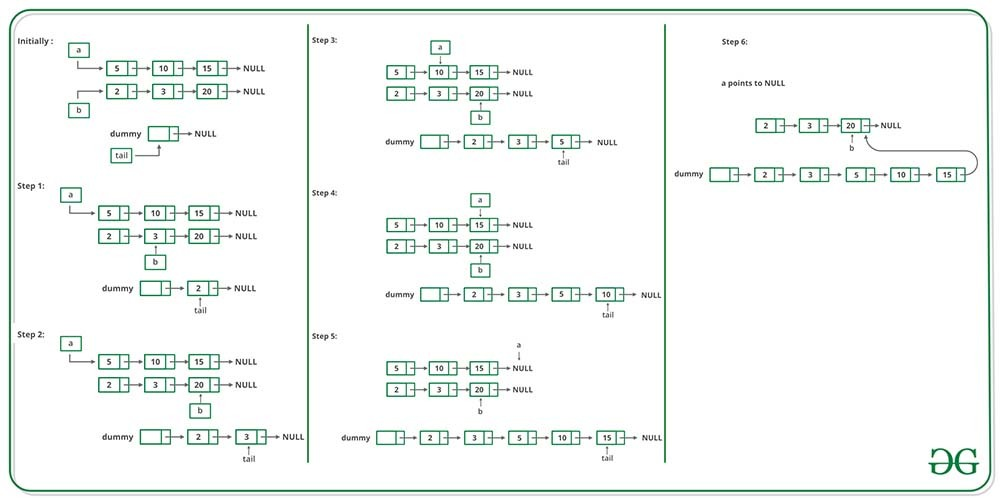## C

 `// C program to merge two sorted``// linked lists``#include ``#include ``#include `` ` `// Linked List Node``struct` `Node {``    ``int` `data;``    ``struct` `Node* next;``};`` ` `/* Pull off the front node of the``   ``source and put it in dest`` ``*/``void` `MoveNode(``struct` `Node** destRef,``              ``struct` `Node** sourceRef);`` ` `/* Takes two lists sorted in increasing order,``   ``and splices their nodes together to make``   ``one big sorted list which is returned.  */``struct` `Node* SortedMerge(``struct` `Node* a, ``struct` `Node* b)``{``    ``/* A dummy first node to hang the``       ``result on */``    ``struct` `Node dummy;`` ` `    ``/* tail points to the last result node  */``    ``struct` `Node* tail = &dummy;`` ` `    ``/* so tail->next is the place to add new``       ``nodes to the result. */``    ``dummy.next = NULL;``    ``while` `(1) {``        ``if` `(a == NULL) {``            ``/* if either list runs out, use the``               ``other list */``            ``tail->next = b;``            ``break``;``        ``}``        ``else` `if` `(b == NULL) {``            ``tail->next = a;``            ``break``;``        ``}``        ``if` `(a->data <= b->data)``            ``MoveNode(&(tail->next), &a);``        ``else``            ``MoveNode(&(tail->next), &b);``        ``tail = tail->next;``    ``}``    ``return` `(dummy.next);``}`` ` `/* UTILITY FUNCTIONS */``/* MoveNode() function takes the node``   ``from the front of the source, and``   ``move it to the front of the dest.``   ``It is an error to call this with the``   ``source list empty.`` ` `   ``Before calling MoveNode():``   ``source == {1, 2, 3}``   ``dest == {1, 2, 3}`` ` `   ``After calling MoveNode():``   ``source == {2, 3}``   ``dest == {1, 1, 2, 3} */``void` `MoveNode(``struct` `Node** destRef,``              ``struct` `Node** sourceRef)``{``    ``/* The front source node  */``    ``struct` `Node* newNode = *sourceRef;``    ``assert``(newNode != NULL);`` ` `    ``/* Advance the source pointer */``    ``*sourceRef = newNode->next;`` ` `    ``/* Link the old dest off the new node */``    ``newNode->next = *destRef;`` ` `    ``/* Move dest to point to the new node */``    ``*destRef = newNode;``}`` ` `/* Function to insert a node at the beginning of the``   ``linked list */``void` `push(``struct` `Node** head_ref, ``int` `new_data)``{``    ``/* allocate node */``    ``struct` `Node* new_node``        ``= (``struct` `Node*)``malloc``(``sizeof``(``struct` `Node));`` ` `    ``/* put in the data  */``    ``new_node->data = new_data;`` ` `    ``/* link the old list off the new node */``    ``new_node->next = (*head_ref);`` ` `    ``/* move the head to point to the new node */``    ``(*head_ref) = new_node;``}`` ` `/* Function to print nodes in a given linked list */``void` `printList(``struct` `Node* node)``{``    ``while` `(node != NULL) {``        ``printf``(``"%d "``, node->data);``        ``node = node->next;``    ``}``}`` ` `/* Driver program to test above functions*/``int` `main()``{``    ``/* Start with the empty list */``    ``struct` `Node* res = NULL;``    ``struct` `Node* a = NULL;``    ``struct` `Node* b = NULL;`` ` `    ``/* Let us create two sorted linked lists to test``      ``the functions``       ``Created lists, a: 5->10->15,  b: 2->3->20 */``    ``push(&a, 15);``    ``push(&a, 10);``    ``push(&a, 5);`` ` `    ``push(&b, 20);``    ``push(&b, 3);``    ``push(&b, 2);`` ` `    ``/* Remove duplicates from linked list */``    ``res = SortedMerge(a, b);`` ` `    ``printf``(``"Merged Linked List is: \n"``);``    ``printList(res);`` ` `    ``return` `0;``}`

Output

```Merged Linked List is:
2 3 5 10 15 20 ```